# Multistage Wilkinsons

Click here to go to our main page on Wilkinson power splitters

Click here to go to our page on designing Wilkinson splitters with Excel

New for September 2014! How many sections do you need in a multi-stage Wilkson? Here's a page that tells you how to know, before you begin the design.

New for December 2012! Here's a link to a UK college web site that is showing students how to design multi-section Wilkinsons, including detailed schematics and artwork. Thanks to Alan. Bravo!

Multistage (or multi-section) Wilkinson power splitters can perform remarkably well over more than a decade of bandwidth. On this page we will show you a procedure that will help you design a multi-section Wilkinson quickly and efficiently, instead of using guesswork and optimization. Then you can cheaply build your own splitters instead of buying them from Narda for thousands of dollars!

Example 2 presents info on the exact design of Chebyshev and maximally flat multistage Wilkinson splitters, using our impedance transformer spreadsheet that you can download! Does Agilent offer this in ADS? Heck no, Dude!

Recently a question came up about what to use as center frequency for a broadband design. Should lambda/4 represent the arithmetic mean (the average), or something else, like the geometric mean. The answer is arithmetic, my dear Watson... for 6 to 18 GHz, the center frequency is 12 GHz, and all the sections should be a quarter-wavelength at 12 GHz. Thanks for asking, Tamas!

### Calculating isolation resistors

For a multistage Wilkinson, we haven't (yet) developed a detailed method for determining isolation resistors along the chain. Harlan Howe's book on stripline design has some nice tables that can help, he also shows some equations. Seymour Cohn wrote an excellent article on the subject way back in 1968, you can download it from IEEE Explore if you are a member. Sorry, we try to respect restrictions on copyrighted material so we won't post the article. Here's the exact reference:

A Class of Broadband Three-Port TEM-Mode Hybrids
Cohn, S.B., Microwave Theory and Techniques, IEEE Transactions on
Volume 19, Issue 2, Feb. 1968 Page(s): 110 - 116

Thanks to Tim for this IEEE reference! Table 1 gives normalized quarter-wave line impedances and resistor values for various bandwidth designs. Cohn does give closed-form equations for the two resistors in a two-stage Wilkinson for equal-ripple response, then shows some approximations for cases higher than N=2. In the end, you will probably have to use an optimizer such as you'll find in Agilent's ADS to squeeze out the maximum isolation over your design bandwidth.

Isolation resistors are sometimes referred to as "dump" resistors, an example of microwave slang. Also thanks to Tim!

New for August 2008! We created a spreadsheet that will allow you to analyze multi-section Wilkinsons in Excel, including isolation between the split ports. It's explained on this page.

### Example 1: designing the hard way...

We put together the two-stage Wilkinson combiner below to illustrate the extra bandwidth that you have achieve with a second set of quarter-wave sections. The values for the line impedances and isolation resistors were obtained by shamelessly using the optimizer in our ancient old copy of Eagleware Superstar... sorry to disappoint anyone that thought we would derive equations for the optimum values!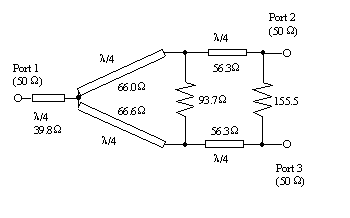Example of two-stage Wilkinson with input transformer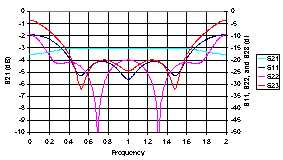S-parameters of above 2-stage Wilkinson with input transformer

### Example 2A, two-stage, 75 ohms, the easy way!

This two stage divider was calculated using our transformer spreadsheet located in the download area. The arm impedance has to be twice that of the transformer solution, because two arms are in parallel.

The circuit is a two stage, Chebyshev Wilkinson, with characteristic impedance 75 ohms (instead of the usual 50 ohms, just for fun).

The required frequency band was 950 to 2025 MHz, because that's what a Microwaves101 reader requested. The center frequency is 1.488 GHz (=(F1+F2)/2). The load and source resistance that the transformer is matching to is 75 ohms (the common port) and 37.5 ohms (the split ports, two 75 ohm loads in parallel). The Chebyshev transformer spreadsheet calculates 61.248 and 45.920 ohms for the two sections; you need to double these values to 122.5 and 91.8 ohms, as we stated before, because the two arms are in parallel. Note that the impedances are a function of the percentage bandwidth as well as the termination impedances. You can view the VSWR of the input port from within the spreadsheet, this is very handy and will help you decide how many sections to use for your application.

The isolation resistors values were found by referring to a graph in Harlan Howe's book "Stripline Circuit Design". You can find this book in our page on microwave engineering books. Note that more than one solution exists for the resistors, depending on what you want to optimize (isolation at center frequency, bandwidth of S22, etc.)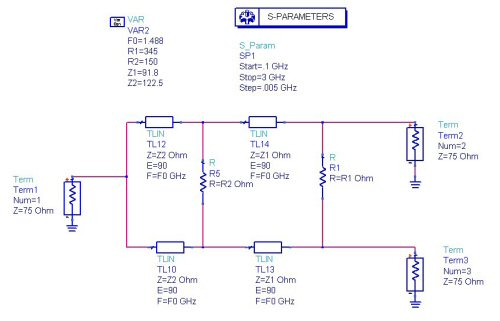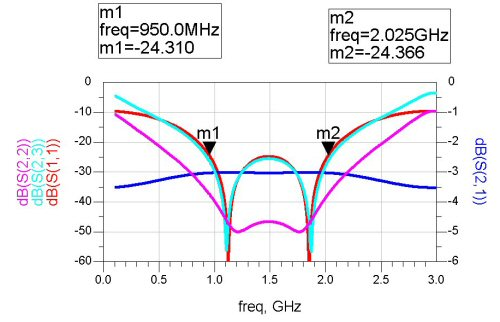### Example 2B, two-stage, 75 ohms, with transformers

In the next example, we added a pair of transformers to reduce the 75 ohm terminations to 50 ohms at each port (61.2 ohm quarter-wave three transformers did the job). This reduces the maximum impedance of the arms, they are now Z1=61.2 and Z2=81.7 ohms (exactly 2/3 what they were before, because we reduced 75 ohms to 50 ohms). Z2 at 122.5 ohms might have been unrealizable previously. The isolation resistors were also scaled by 2/3 from the previous example. The resulting input impedance (S11) stays close to equal-ripple in the passband.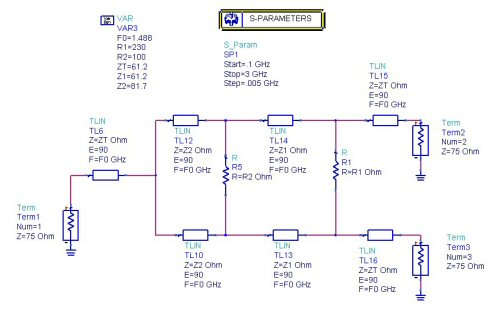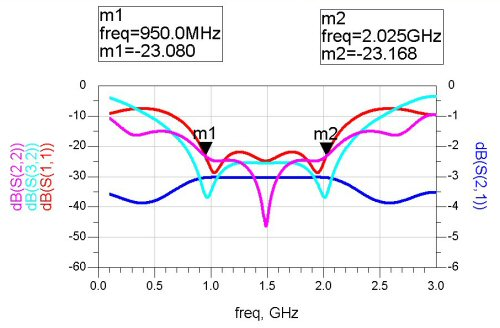### Example 2C: 950 to 2025 MHz, three stages

Below we designed a three stage Wilkinson, this time with a "physical" model. The transmission lines are microstrip on Rogers 4003 (ER=3.38), 32 mils thick with half-ounce copper (0.7 mils). Again, we are looking for 950 to 2025 MHz bandwidth, but we want more isolation (hence three stages). We used the starting values for line impedances from our transformer and microstrip calculators, took the isolation resistor values from Harlan Howe's book, then did a little optimization using Agilent ADS. The next two plots show the model and predicted performance. We rounded off the resistors to 1% RETMA values. The isolation that you get will probably be six dB worse than the prediction (which was greater than 30 dB), because the resistors won't act ideal.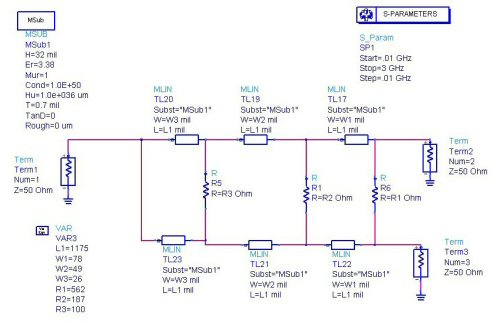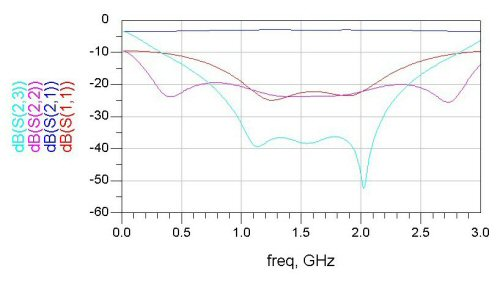### Example 3

Yet another request from the Microwaves101 message board. This is for 800 MHz to 6 GHz. We arbitrarily chose four sections because we don't have a specification to go by.

If you download the transformer calculator to use as a multisection Wilkinson 2-way design, we recommend you start with the equal ripple page, enter input impedance = 50 ohms, output=25 ohms (Z0/N, where N=number of outputs...) for a three-way, output=16.7 ohms etc.

Then enter the frequency range, 0.8 to 6 GHz for this example. The number of sections can be traded for VSWR (more sections=lower VSWR ripples).

The calculator will give you the section impedances, for example if you have a two-way divider (50:25 ohms) with four sections, the transformer spreadsheet will give you these impedances:

41.243
37.299
33.513
30.308

These you will use as the arms for the Wilkinson. But first you have to double them because the two arms will be in parallel. The low values go toward the 2-way side, the high values toward the 50 ohms side.

The "magic" you will have to perform on your own is to calculate isolation resistors,

Next we simulated the Wilkinson on ADS, and used 200 ohm isolation resistors. Why 200 ohms? It's a pidooma!

Here's the schematic and the response: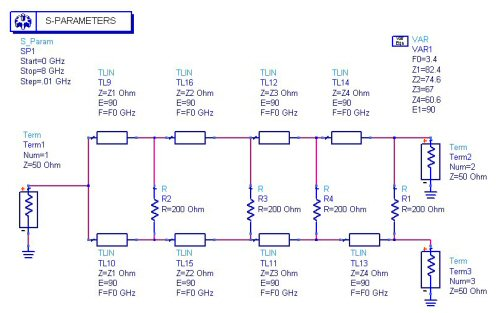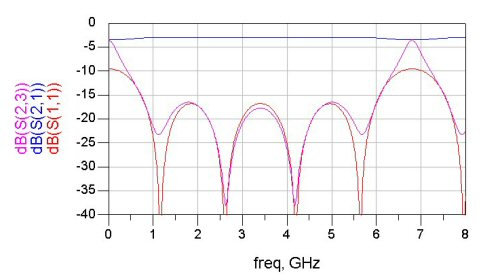The isolation is pretty good at 17 dB, but it could be better.

We used the ADS optimizer and came up with 20 dB isolation across the band: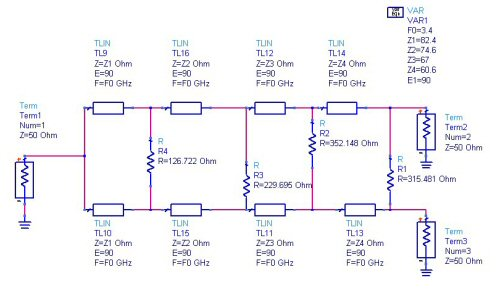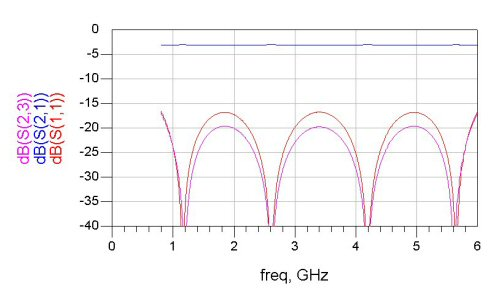That took all of 15 minutes!

Here's the group delay of the splitter: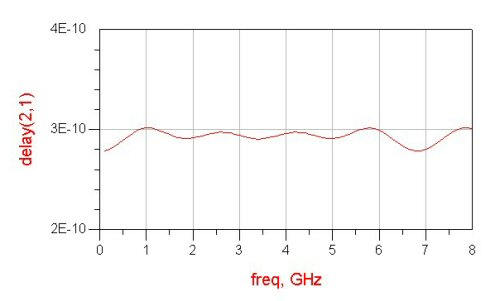Coming soon! Maybe a layout of a Wilkinson, and maybe some EM analysis!

Author : Unknown Editor